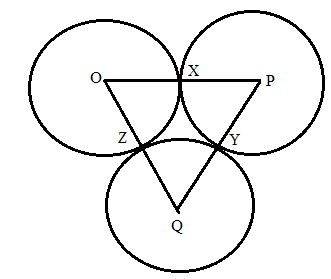Chapter 6.3, Problem 43EElementary Geometry For College St...

7th Edition
Alexander + 2 others
ISBN: 9781337614085

Solutions

Chapter
SectionElementary Geometry For College St...

7th Edition
Alexander + 2 others
ISBN: 9781337614085
Textbook Problem

Circles O, P, and Q are tangent (as shown for Exercise 40).If O X = 3 , P Y = 4 , Q Z = 1 , name:a) .The smallest angle of Δ P Q O b) .The smallest angle of Δ P Q OTo determine

a)

To find:

To name the smallest angle of ΔPQO if:

OX=2,PY=3,QZ=1

Explanation

Given that, circles O, P, and Q are tangent (as shown) at points X, Y, and Z.

Then the value of OX=3,PY=4,QZ=1.

The diagrammatic representation is given below,

To find the measures of the sides of the triangle ΔPQO is given below,

OP=OX+PXPQ=PY+QYOQ=QZ+OZ

We know that OX=OZ,PX=PY,QY=QZ since radius of the circle is same for all points on the circle.

Substitute the value of OX=3,PY=4,QZ=1 and OX=OZ,PX=PY,QY=QZ to get the following,

O<

To determine

b)

To find:

To name the largest angle of ΔPQO if:

OX=2,PY=3,QZ=1

Still sussing out bartleby?

Check out a sample textbook solution.

See a sample solution

The Solution to Your Study Problems

Bartleby provides explanations to thousands of textbook problems written by our experts, many with advanced degrees!

Get Started

If f(x)=x+x, show that lim x0 f(x) exists hut is not equal to f(2).

Single Variable Calculus: Early Transcendentals, Volume I

Let f be the function defined by f(x)={2+1xifx111xifx1 Find f(0), f(1), and f(2).

Applied Calculus for the Managerial, Life, and Social Sciences: A Brief Approach

30. For what value of c is the function a probability density function?

Mathematical Applications for the Management, Life, and Social Sciences

Evaluate the integral. 19. z3ezdz

Calculus: Early Transcendentals